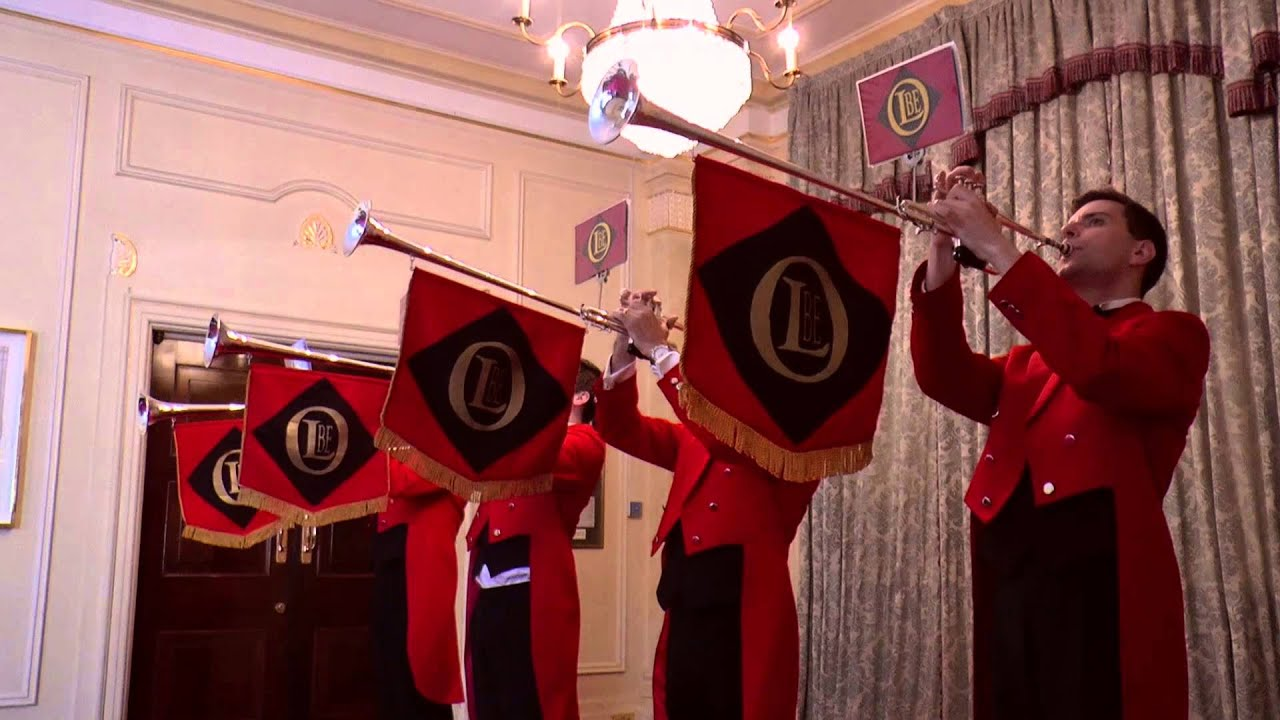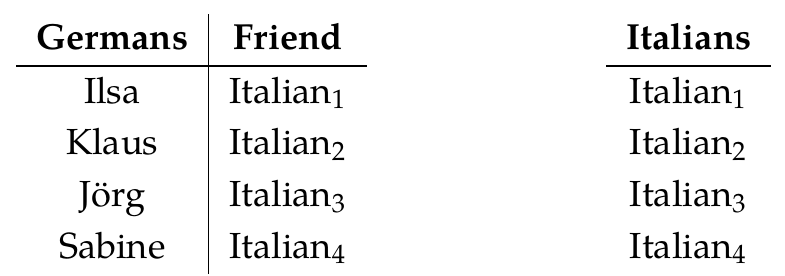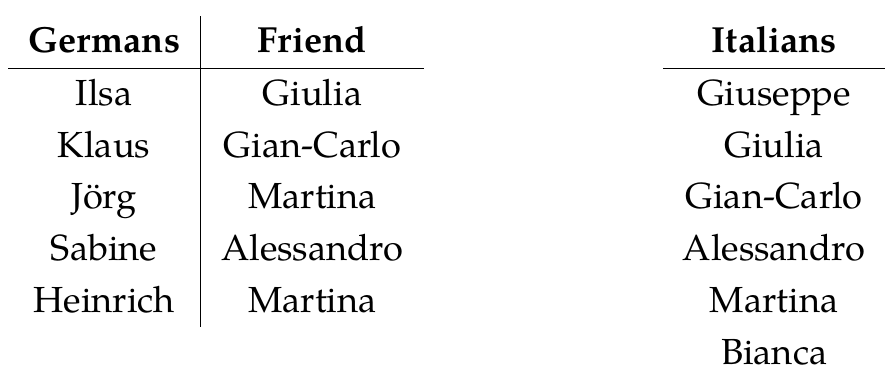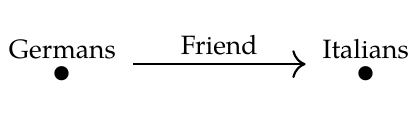So, on to the main puzzles, Puzzles 155-158!I think you folks have mostly done them, but there's a bit left to do, and it's important for everyone to think about these, so let me start working through them very slowly and systematically - and everyone who has questions or comments jump in!

> **Puzzle 155.** Describe a natural transformation \$$\alpha : \textrm{Lan}_G(H) \Rightarrow F\$$ where \$$\textrm{Lan}_G(H) \$$ is this database:

>> and \$$F\$$ is this database:

>Both \$$\textrm{Lan}_G(H)\$$ and \$$F\$$ are functors from \$$\mathcal{C}\$$ to \$$\mathbf{Set}\$$, where \$$\mathcal{C}\$$ is the free category on this graph:So, a natural transformation \$$\alpha : \textrm{Lan}_G(H) \Rightarrow F\$$ consists of functions

$\alpha_{\text{Germans}} : \textrm{Lan}_G(H)(\text{Germans}) \to F(\text{Germans})$

and

$\alpha_{\text{Italians}} : \textrm{Lan}_G(H)(\text{Italians}) \to F(\text{Italians}) .$

Christopher proposed a choice for both of these:

> Well let's be a bit silly and take all the Germans to Heinrich, then naturality forces us to take all the Italians to Martina.

By staring at the table above, note that

$\textrm{Lan}_G(H)(\text{Germans}) = \\{ \text{Ilsa}, \text{Klaus}, \mathrm{Jörg}, \text{Sabine} \\}$

and

$F(\text{Germans}) = \\{ \text{Ilsa}, \text{Klaus}, \text{Jörg}, \text{Sabine}, \text{Heinrich} \\}.$

So, we need to choose a function

$\alpha_{\text{Germans}} : \\{ \text{Ilsa}, \text{Klaus}, \text{Jörg}, \text{Sabine} \\} \to \\{ \text{Ilsa}, \text{Klaus}, \text{Jörg}, \text{Sabine} , \text{Heinrich} .\\}$

Christopher seems to be hinting that we can take this to be _any_ function, and that naturality then _forces_ a specific choice of the function

$\alpha_{\text{Italians}} : \textrm{Lan}_G(H)(\text{Italians}) \to F(\text{Italians}) .$

That's true. The key is the commutative square in the definition of [natural transformation](https://forum.azimuthproject.org/discussion/2244/lecture-43-chapter-3-natural-transformations/p1)!

Could someone show, in a bit of detail, how this works? Some people instinctively 'get' the power of naturality. Others don't. My job is to make people get it, who don't yet. Maybe someone who is just starting to get it would enjoy filling in the details here!